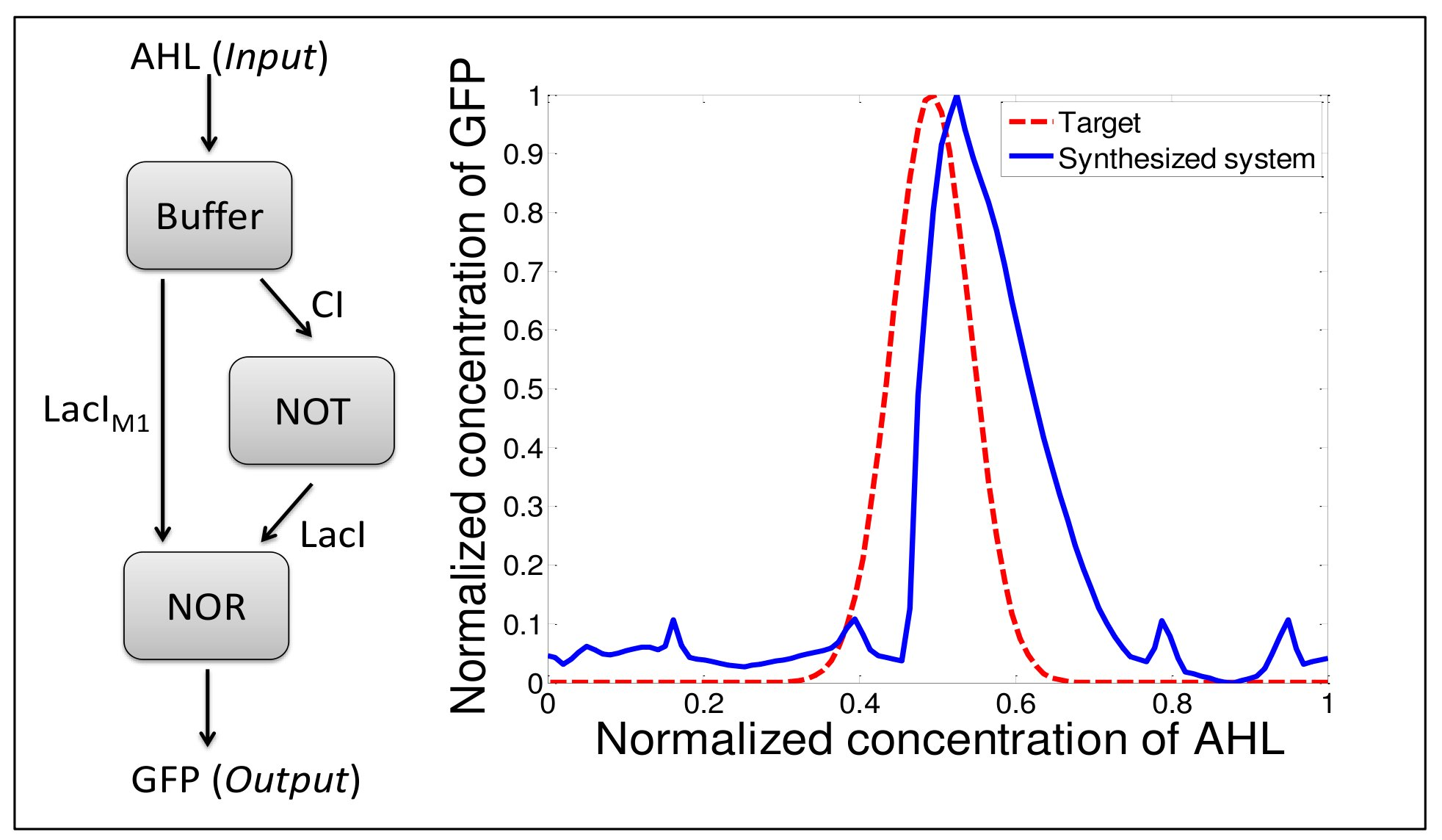Design of artificial gene regulation network with fuzzy logic approach

Morgan MADEC, Jacques HAIECH, Christophe LALLEMENT, Yves GENDRAULT

Telecom Physique Strasbourg, Illkirch, FR
 Fuzzy logic is a branch of mathematics introduced in 1965 by Zadeh1. It is a kind of multi-valued logic where inputs and outputs of the systems are continuous values but the behavior of the system is described by a finite number of Boolean rules (matrix of rules). In this tutorial, we aim at demonstrating that fuzzy logic can be a helpful tool for the design and the modeling of artificial gene regulation network2. The tutorial can be divided in four part and use as illustration a AHL band detector developed in 2005 by Basu et al3 (on the left of the figure). 1/ Theoretical approach The first part is a theoretical approach in which the concepts of fuzzy logic are described. It consists in a short lecture and a paper exercise in which the model of one gene of the band detector is hand-computed. 2/ Simulation software In the second part, we introduce a software, designed by our team, that simulates a genetic network thru fuzzy logic. The software is encoded in C and the matrix of rules is specified in a text file. Some exercises are proposed to the attendees in order to familiarize with this software. 3/ Use of the software for the design of the band detector In the third part, the fuzzy simulator is used to design the band detector according to a targeted dose-response curve (red curve on the figure). For the three genes of the library of various matrix of rules are proposed. The user computes, through the simulator, the dose-response curve for each combination of matrix of rules and chooses, according to a given criterion, which of them is the closest to the target. According to this result, the user can draw several properties of the genes to implement in order to achieve the desired result (the affinity of regulating proteins with the promoter, cooperativity between binding sites on the promoter ...) 4/ Simulation of the resulting construct with COPASI In the last step of the tutorial, the accuracy of the fuzzy model is compared with the simulation results that can be obtained with standard (ordinary differential equation model). For this purpose, software that converts a fuzzy description into a SBML description has been developed. The SBML description uses standard transcription and translation models and the parameters of the model (transcription and translation rates, regulation protein affinity, Hill's parameters) are fixed to a default value according to the corresponding matrix of rules. The SBML description can be simulated with COPASI. Some alternatives (using MATLAB or python) can be provided as a function of attendee's habits.References

 1 L.A. Zadeh, "Fuzzy Sets", Information and Control, vol. 8, pp. 338-353, 1965. 2 Y. Gendrault et al. " Fuzzy logic, an intermediate description level for design and simulation in synthetic biology", Proc. IEEE BioCAS, Rotterdam (NL), 30 Oct Ł 2 Nov 2013. 3 S. Basu et al., "A synthetic multicellular system for programmed pattern formation", Nature, vol. 434, 2005.